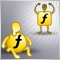Custom indicator inside an EA : Array out of range11611

Hi,

Here's a the code that cause an error in an indicator I use :

datetime tm=time[rates_total-20];

The error is :

array out of range in 'pivot_lines.mq5' (428,20)

May someone help ?

EDIT : I've change the Max Bar in chart parameters to 50 000, it was using 100 000 - just to try  if it's a memory lack ... :/Documentation on MQL5: Standard Constants, Enumerations and Structures / Environment State / Client Terminal Properties
• www.mql5.com
Standard Constants, Enumerations and Structures / Environment State / Client Terminal Properties - Reference on algorithmic/automated trading language for MetaTrader 539020

blouf:

Hi,

Here's a the code that cause an error in an indicator I use :

The error is :

May someone help ?

EDIT : I've change the Max Bar in chart parameters to 50 000, it was using 100 000 - just to try  if it's a memory lack ... :/

It probably shouldn't. Can you show this line of code in a more general context ?

Did you get this error systematically, or only in some circumstances ?11611

angevoyageur:

It probably shouldn't. Can you show this line of code in a more general context ?

Did you get this error systematically, or only in some circumstances ?

I never get it elsewhere than on the VPS server. I never saw it in Strategy Tester, nor in live, it happens on many symbols but only when running on the vps

for(int i=start;i<rates_total;i++){
BufHigh[i]=BufHigh[i-1];
BufLow[i]=BufLow[i-1];

PivotBuffer[i]=PivotBuffer[i-1];
S1Buffer[i]=S1Buffer[i-1];
R1Buffer[i]=R1Buffer[i-1];
S2Buffer[i]=S2Buffer[i-1];
R2Buffer[i]=R2Buffer[i-1];
S3Buffer[i]=S3Buffer[i-1];
R3Buffer[i]=R3Buffer[i-1];

M0Buffer[i]=M0Buffer[i-1];
M1Buffer[i]=M1Buffer[i-1];
M2Buffer[i]=M2Buffer[i-1];
M3Buffer[i]=M3Buffer[i-1];
M4Buffer[i]=M4Buffer[i-1];
M5Buffer[i]=M5Buffer[i-1];

L3Buffer[i]=L3Buffer[i-1];
H3Buffer[i]=H3Buffer[i-1];
L4Buffer[i]=L4Buffer[i-1];
H4Buffer[i]=H4Buffer[i-1];

datetime NowTime=time[i]-TimeShift;
datetime PreTime=time[i-1]-TimeShift;

if(NewDay(NowTime,PreTime)){
if(BufHigh[i]!=0){
P=(BufHigh[i]+BufLow[i]+close[i-1])/3;
R1=(2.0*P)-BufLow[i];
S1=(2.0*P)-BufHigh[i];
R2=P+(BufHigh[i]-BufLow[i]);
S2=P-(BufHigh[i]-BufLow[i]);
R3=2.0*P+(BufHigh[i]-2.0*BufLow[i]);
S3=2.0*P-(2.0*BufHigh[i]-BufLow[i]);

if(PivotsBufers){
PivotBuffer[i]=P;
S1Buffer[i]=S1;
R1Buffer[i]=R1;
S2Buffer[i]=S2;
R2Buffer[i]=R2;
S3Buffer[i]=S3;
R3Buffer[i]=R3;
}

Q=(BufHigh[i]-BufLow[i]);
H4=(Q*0.55)+close[i-1];
H3=(Q*0.27)+close[i-1];
L3=close[i-1]-(Q*0.27);
L4=close[i-1]-(Q*0.55);

if(CamarillaBuffers){
L3Buffer[i]=L3;
H3Buffer[i]=H3;
L4Buffer[i]=L4;
H4Buffer[i]=H4;
}

M5=(R2+R3)/2;
M4=(R1+R2)/2;
M3=(P+R1)/2;
M2=(P+S1)/2;
M1=(S1+S2)/2;
M0=(S2+S3)/2;

if(MidpivotsBuffers){
M0Buffer[i]=M0;
M1Buffer[i]=M1;
M2Buffer[i]=M2;
M3Buffer[i]=M3;
M4Buffer[i]=M4;
M5Buffer[i]=M5;
}

}
BufHigh[i]=high[i];
BufLow[i]=low[i];
}
else{
BufHigh[i]=MathMax(BufHigh[i],high[i]);
BufLow[i]=MathMin(BufLow[i],low[i]);
}
}

datetime tm=time[rates_total-20];      <------------- the line

if(PivotsLines){
fObjMove("Pivot",P,tm);
fObjMove("S1",S1,tm);
fObjMove("R1",R1,tm);
fObjMove("S2",S2,tm);
fObjMove("R2",R2,tm);
fObjMove("S3",S3,tm);
fObjMove("R3",R3,tm);11611

blouf:

I never get it elsewhere than on the VPS server. I never saw it in Strategy Tester, nor in live, it happens on many symbols but only when running on the vps

But I could ignore this error if I was sure it doesn't interrupt the EA39020

blouf:
But I could ignore this error if I was sure it doesn't interrupt the EA

You get this error when using the indicator with iCustom/CopyBuffer ?

If yes, it's a fatal error, it interrupts your EA.11611

angevoyageur:

You get this error when using the indicator with iCustom/CopyBuffer ?

If yes, it's a fatal error, it interrupts your EA.

Yeah, that's how I use it. Is there anyway to correct this ?39020

blouf:

I never get it elsewhere than on the VPS server. I never saw it in Strategy Tester, nor in live, it happens on many symbols but only when running on the vps

The only reason I can see is if rates_total is lesser than 20. Problem with data on your VPS.11611

angevoyageur:
The only reason I can see is if rates_total is lesser than 20. Problem with data on your VPS.

Maybe the memories then, I can't see how it can have less than 20 candles. I will try with the workaround I talked about above, if not good I will remove it.

But tell me please, if it's a fatal error, how to reload it ?39020

blouf:

Maybe the memories then, I can't see how it can have less than 20 candles. I will try with the workaround I talked about above, if not good I will remove it.

But tell me please, if it's a fatal error, how to reload it ?

Add error checking to avoid the error.11611

angevoyageur:
Add error checking to avoid the error.

I have :

- Reduced the Maxbar in chart properties

- Add error checking

- And decreased memories consumption using this post :

https://www.mql5.com/en/articles/259

Let's wait & see.Decreasing Memory Consumption by Auxiliary Indicators
• 2011.06.02
• Andrew
• www.mql5.com
If an indicator uses values of many other indicators for its calculations, it consumes a lot of memory. The article describes several methods of decreasing the memory consumption when using auxiliary indicators. Saved memory allows increasing the number of simultaneously used currency pairs, indicators and strategies in the client terminal. It increases the reliability of trade portfolio. Such a simple care about technical resources of your computer can turn into money resources at your deposit.